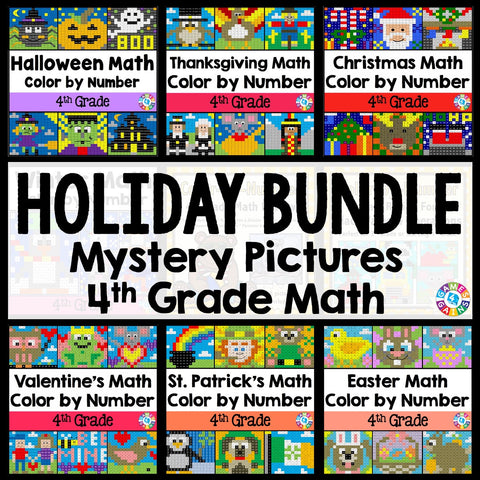## 4th Grade Math Color-by-Number Holiday Bundle

• \$3400
• Save \$8.45

This 4th Grade Math Color-by-Number Holiday Bundle comes to you after receiving numerous requests from buyers to create a bundle that includes all of my seasonal 4th grade math color-by-number sets!

This HUGE bundle covers a wide variety of 4th grade math skills and includes 52 mystery pictures for celebrating all of the major holidays during the school year. Students will have a blast each holiday as they practice key math skills in a fun and COLORFUL way!

Plus, you will SAVE \$\$\$ when you purchase this bundle. If you bought each holiday set individually, you would spend \$40.

Want to learn more about what's included in this bundle? Click on the links below to view the individual previews for each activity. Remember, you'll get all of these holiday sets when you purchase this bundle!

"School Days" Place Value and Operations Color-by-Number Activities (3rd/4th Grade)

*Note: This set is a REVIEW of 3rd grade math skills, so that 4th grade teachers can use these to review with their incoming 4th graders.

• Rounding numbers to the nearest 10 and 100
• Finding the unknown in basic multiplication facts
• Multiplying one-digit whole numbers by multiples of 10 (10-90).
• Solving basic division facts
• Subtracting within 1,000

Halloween Math Color-by-Number Activities (4th Grade)

• Writing numbers within 1,000,000 in standard form from expanded form and word form
• Comparing numbers within 1,000,000
• Rounding numbers within 1,000,000
• Adding and subtracting numbers within 1,000,000 using the standard algorithm
• Adding and subtracting numbers within 1,000,000 in one-step word problems
• Adding and subtracting numbers within 1,000,000 in two-step word problems

Thanksgiving Math Color-by-Number Activities (4th Grade)

• Ordering numbers within 1,000,000
• 1,000/10,000/100,000 more or less (with numbers written in standard, word, and expanded form)
• Adding and subtracting numbers within 1,000,000
• Subtracting larger numbers across zeros
• Solving multiplicative comparison statements (i.e. 24 is ___ times as many as 4)
• Finding the remainder of basic division facts (2-digit by 1-digit)

Christmas Math Color By Number (4th Grade)

• Identifying factors, multiples, and prime/composite numbers
• Understanding place value (the digit to the left is 10 times the underlined digit)
• Multiplying larger numbers (up to 4-digit x 1-digit and 2-digit x 2-digit)
• Dividing larger numbers (up to 4-digit by 1-digit, no remainders)
• Identifying equivalent fractions (denominators 2, 3, 4, 5, 6, 8, 10, 12 only)
• Calculating perimeter and area

Winter Math Color By Number (4th Grade)

• Multiplying large numbers by 10 word problems
• Rounding large numbers word problems
• Adding and subtracting large numbers word problems
• Multiplying and dividing numbers word problems (up to 2-digit multiplied by 2-digit and 4-digit divided by 1-digit)

Valentine's Day Math Color-by-Number Activities (4th Grade)

• Interpreting remainders in word problems
• Multiplying 2-digit by 2-digit numbers
• Adding and subtracting proper fractions with like denominators (no simplifying answers, but answers may be improper fractions that must be turned into a mixed number)
• Comparing fractions with unlike denominators (denominators 2, 3, 4, 5, 6, 8, 10, 12)
• Converting units of time from a larger unit to a smaller unit
• Identifying prime and composite numbers between 0 and 40

St. Patrick's Day Math Color-by-Number Activities (4th Grade)

• Adding and subtracting mixed numbers with like denominators
• Generating a number pattern from a one-step rule
• Converting customary measurements of capacity (larger unit to smaller unit)
• Calculating the perimeter of an irregular figure (including finding missing side dimensions)
• Measuring angles to the nearest degree
• Identifying points, rays, lines, line segments, and types of angles

Easter Math Color-by-Number Activities (4th Grade)

• Solving multiplicative comparison problems with multiples of 10
• Multiplying proper fractions by whole numbers
• Writing decimals from decimal models
• Converting customary lengths from the larger unit to the smaller unit (yards, feet, inches)
• Calculating area
• Determining the missing angle measurement

Summer Math Color-by-Number Activities (4th Grade)

• Adding, subtracting, and multiplying fractions (proper fraction x whole number)
• Multiplying and dividing larger numbers
• Determining the factors and multiples of a given number
• Converting units of measurement
• Calculating perimeter and area
• Measuring angles